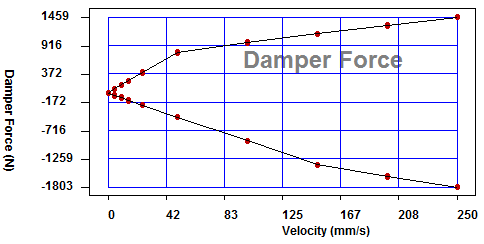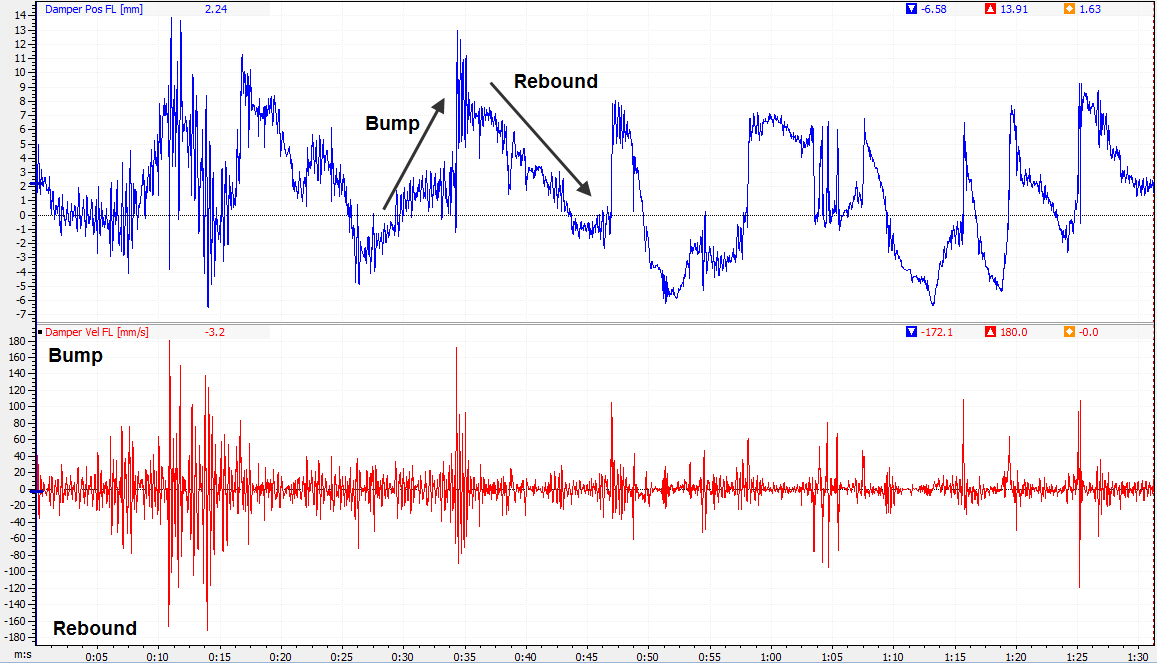# Tuning shock absorbers using the shock speed histogram

 Tuning shock absorbers using the shock speed histogram The shock speed histogram is a useful method to characterize race car shock absorber characteristics and performance from data recorded by the onboard data logger. This paper covers shows the reader how to use shock speed histograms to learn more about shock absorber behaviour and how to use these diagrams to tune them. Introduction The purpose of a shock absorber on a race car is twofold: to minimize the variation between the tire’s contact patch and the track surface, but also to influence the transient chassis motions as the car is subjected to lateral, longitudinal and vertical acceleration. Shock absorbers are speed sensitive. They develop a force which is proportional to the speed at which they compress or extend. When you compress a shock absorber slowly, the resisting force will be smaller than when you try to compress it faster. As a comparison, springs develop a force proportional to the distance they’re compressed or extended, regardless of the speed of this movement. Basically, this means that a spring develops its maximum force at maximum deflection whereas a shock absorber reaches its maximum force there where the shaft speed is at a maximum. The characteristic of any given shock absorber can be tested on a shock dynamometer. This will typically give you a shock force versus shaft speed as illustrated in Figure 1. A graph such as this will show you which force is developed at a particular speed and this for compression and extension (bump or rebound).Figure 1 Shock force versus shaft speed. Shock speed The first step in shock absorber tuning is to determine the characteristic that is in the shock absorber. For this we need to test it on a dynamometer to obtain the shock force versus shaft speed curve. Next we have to look at which speeds a shock absorber is subjected to as the car travels down the racetrack. This we can determine from recorded suspension data. Linear potentiometers are typically used to measure suspension movement. When the potentiometer is placed in line with the shock absorber we’re measuring shock absorber movement. If this is not the case then there’s a certain motion ratio between the signal of the potentiometer and the shock movement. This motion ratio can easily be determined by removing the spring from the suspension and jacking the wheel up and down. A simple mathematical channel allows us to calculate the shock speed by taking the first derivative of the shock movement channel. When we illustrate both channels as a function of time we will see something resembling the graph in Figure 2.Figure 2 Shock absorber movement and speed logged data. The upper trace in Figure 2 shows us the damper travel along a lap around the racetrack. An increasing trend in this graph corresponds to compression of the shock absorber (bump), while a decreasing trend indicates that the shock absorber is in extension (rebound). In the lower trace, the shock speed, this means that a positive value indicates bump, while a negative value means rebound. A quick look at this trace shows us a small amount of peaks into the region of ±180 mm/s, while the bulk of the shock speed is located between ±20 mm/s. Generally the low speed range of the shock absorber will influence the transient handling of the car when it is subjected to lateral, longitudinal and vertical acceleration, while the high speed range takes care of road input. For the sake of argument, we will distinguish the following shock speed ranges: